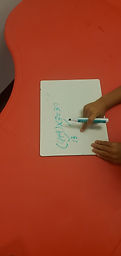Mr. Jake

Target 1​

Lesson Type:

Continuation

Measurment

:

Unit Conversion

Convert measurement data within a given measurement system.

1:

Understand equivalent measurements.

2:

Understand how to convert from one unit to another.

3:

Use scale to reproduce a figure at either a bigger or smaller size (relate the measurement of the original figure to the new figure).

5th

Vocabulary:

Fraction, Unit, Convert

Activities:

Measured objects using fractional measurments

Converted between different types of units using fractional measurementsHome Exploration

Guiding Questions:Absent Students:

Target 2

:

1:

Solve problems that have more than one operation.

2:

Understand that the presence of parenthesis in an arithmetic equation signals for this part of the problem to be solved first.

3:

Understand that the order of operations must be followed to evaluate expressions.

4:

Understand that calculating in the wrong order can result in a wrong answer.

5:

Define PEMDAS – Parenthesis, Exponents, Multiplication, Division, Addition, Subtraction.

6th

Vocabulary:

Order of Operations, PEMDAS

Activities:

Practiced using the order of operations to solve problemsHome Exploration

Guiding Questions:Target 3

:

Vocabulary:

Activities:Home Exploration